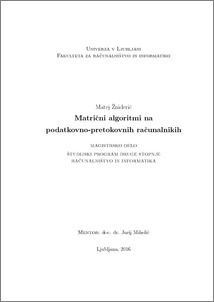# Matrix algorithms on data-flow computers

Matej Žniderič (2017) Matrix algorithms on data-flow computers. MSc thesis.Preview
PDF

## Abstract

While the processor frequency has failed to significantly increase in the last decade, the needs of science for computing power continue to increase. Data-flow computer architecture presents a good alternative to traditional control-flow computer. In this work a set of algorithms for matrix-vector multiplication and matrix-matrix multiplication was developed. It was compared with control-flow implementations. With matrix-vector multiplication there was no speedup. Algorithm for multiplying matrix with a set of vectors achieved almost 4-fold speedup. Matrix-matrix multiplication algorithm achieved more than 100-fold speedup. Further matrix exponentiation and all-pairs shortest path search algorithm were implemented. First algorithm achieved 100-times speedup, while the second one was about the same speed as control-flow Floyd-Warshall algorithm.

Item Type: Thesis (MSc thesis)
Keywords: dataflow architecture, matrix multiplication, matrix algorithms, graph algorithms, Maxeler Technologies
Number of Pages: 86
Language of Content: Slovenian
Mentor / Comentors:
Name and SurnameIDFunction
doc. dr. Jurij Mihelič305UNSPECIFIED